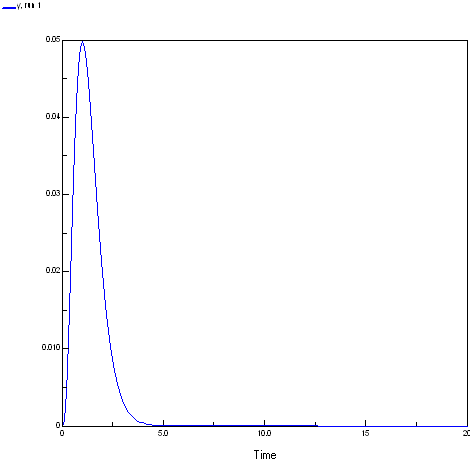# Optimizing model output

This is a simple example of optimizing output of a Simile model using SimileScripting and the Tcllib optimization package math::optimize. The script illustrates how to tackle this kind of problem.

"The package math::optimize is fully implemented in Tcl and no particular attention has been paid to the accuracy of the calculations. Instead, the algorithms have been used in a straightforward manner."

The script uses the procedure `::math::optimize::min_bound_1d` that miminizes a function of one variable in the given interval. The procedure uses Brent's method of parabolic interpolation, protected by golden-section subdivisions if the interpolation is not converging. No guarantee is made that a global minimum is found. In the script the proceedure `func` is defined for `::math::optimize::min_bound_1d` to evaluate. Proc `func` wraps up some SimileScript code that sets the input parameter value, resets the model and then runs the models. Finally it returns the value of a Simile model value for procedure `::math::optimize::min_bound_1d` to minimize.

The package math::optimize also contains the procedure `::math::optimize::nelderMead` that minimizes, in unconstrained fashion, a function of several variable over all of space, which may be worth experimenting with.

### The model

```Model mathOptimize
Enumerated types: []

Variable   x
x = 		Variable parameter
Minimum = 0, Maximum = 10

Variable   y
y = 		x^3* exp(-3.0*x)```

The response function of the model is shown below. To produce the plot the x values were set to the current simulation time.### The script

This script optimises the model output at the end of a run varying one input parameter. The script depends on math::optimize and uses the Plotchart package for a simple plot of the function output for a regular series of inputs the easiest way to get these package is probably to install ActiveTcl.

http://www.activestate.com/products/activetcl/

```# This works to optimise for the last value of models runs.
# The model is run for a set period (SetExecuteFor) and a parameter is set
# to a given value at the start of the run.
#

# this script depends on math::optimize and uses the Plotchart for a
# simple plot of the function output for a regular series of inputs
# the easiest way to get these package is probably to install ActiveTcl
# http://www.activestate.com/products/activetcl/

# Where the extra libraries live MODIFY FOR YOUR CASE	lappend auto_path {c:/program files/tcl/lib}; # eg ActiveTcl directories
package require math::optimize
package require Plotchart

# Simulistics provided package
package require SimileAutoObj; # not actually needed in Windows using SimileScript.exe

# Create a Tcl object/command (modelWin) with which to control that instance of Simile
similescript::ModelWindow modelWin

# Tell Simile not to use the single window Model Run Environment
# We are not going to use any helper anyway here.
# We then load the model and build it using C++
modelWin UseMRE false
# MODIFY FOR YOUR CASE      MUST BE FORWARD SLASHES EVEN UNDER WINDOWS!	modelWin Open "H:/mathOptimize.sml"
modelWin Run

# Create a runControl command/object with which to control
# (as you might expect) the run control.
similescript::RunControl runControl

runControl SetExecuteFor 1
runControl SetTimeStep 1 1

# Create proc to run model and return value of variable to optimize
proc func {x} {
runControl SetValue /x \$x
runControl Reset
runControl Start
# multiply the value by -1 as we want the maximum value using a minimising optimiser
set y [runControl GetValue /y]
return [expr -1*\$y]
}

package require Plotchart

toplevel .t
canvas .t.c -background white -width 400 -height 200
pack   .t.c -fill both

#
# Create the plot with its x- and y-axes
#
set s [::Plotchart::createXYPlot .t.c {0.0 20.0 2.0} {0.0 0.05 0.01}]
\$s title "Shape of function we are evaluating"

# evalute the model output for a range of input values ()
# by plotting the output value for a series of input values
# # multiply the value by -1 to get the original sign
puts "Function shape"
for {set i 0} {\$i<=20} {incr i } {
set x [expr {\$i/2.0}]
set y [expr {-1*[func \$x]}]
puts "\$x \$y"
\$s plot series1 \$x \$y
}

#puts "Maximum at(negative y value): [::math::optimize::min_bound_1d func 0.0 10.0]"
set results [::math::optimize::min_bound_1d func 0.0 10.0 -trace on]
set x [lindex \$results 0]
set y [expr {-1*[lindex \$results 1]}]
puts #####################################################
puts {::math::optimize::min_bound_1d func 0.0 10.0}
puts "Maximum at: \$x = \$y"
puts #####################################################
puts ""

set results [::math::optimize::min_unbound_1d func 0.2 0.7 -trace on]
set x [lindex \$results 0]
set y [expr {-1*[lindex \$results 1]}]
puts {::math::optimize::min_unbound_1d func 0.2 0.7}
puts #####################################################
puts "Maximum at: \$x = \$y"
puts #####################################################```

### Results

You can see its search for the minimum if the trace is on:

```(Run) 159 % ::math::optimize::min_bound_1d func 0.0 10.0 -trace on
f(3.8196601125) = -0.000588169133818 (initialisation)
f(6.1803398875)=-2.09302565156e-006 (golden section)
f(2.360679775)=-0.0110514849929 (golden section)
f(1.4589803375)=-0.039018178181 (golden section)
f(0.901699437495)=-0.0490203182137 (golden section)
f(0.178385003365)=-0.0033239934159 (parabolic interpolation)
f(0.625417908211)=-0.0374683267348 (golden section)
f(1.05516631149)=-0.0495683007526 (parabolic interpolation)
f(1.01193600577)=-0.0497765137855 (parabolic interpolation)
f(1.00189882668)=-0.0497867994449 (parabolic interpolation)
f(0.99971913441)=-0.0497870624755 (parabolic interpolation)
f(0.999993651474)=-0.0497870683649 (parabolic interpolation)
f(1.00000018344)=-0.0497870683679 (parabolic interpolation)
f(0.999999991611)=-0.0497870683679 (parabolic interpolation)
f(0.999999891511)=-0.0497870683679 (parabolic interpolation)
f(0.999997508029)=-0.0497870683674 (golden section)
f(0.999998981102)=-0.0497870683678 (golden section)
f(0.999999543765)=-0.0497870683678 (golden section)
f(0.999999758684)=-0.0497870683679 (golden section)
f(0.999999658584)=-0.0497870683679 (golden section)
0.999999658584 -0.0497870683679```

Maximum at: 0.999999658584 = 0.0497870683679

Page type: